Home

# FINITE-DIMENSIONAL ORDERED VECTOR SPACES WITH RIESZ INTERPOLATION AND EFFROS–SHEN’S UNIMODULARITY CONJECTURE

## Abstract

It is shown that, for any field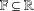$\mathbb{F}\subseteq \mathbb{R}$ , any ordered vector space structure of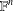$\mathbb{F}^{n}$ with Riesz interpolation is given by an inductive limit of a sequence with finite stages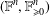$(\mathbb{F}^{n},\mathbb{F}_{\geq 0}^{n})$ (where$n$ does not change). This relates to a conjecture of Effros and Shen, since disproven, which is given by the same statement, except with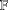$\mathbb{F}$ replaced by the integers,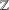$\mathbb{Z}$ . Indeed, it shows that although Effros and Shen’s conjecture is false, it is true after tensoring with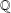$\mathbb{Q}$ .

## References

Hide All
MathJax
MathJax is a JavaScript display engine for mathematics. For more information see http://www.mathjax.org.

# FINITE-DIMENSIONAL ORDERED VECTOR SPACES WITH RIESZ INTERPOLATION AND EFFROS–SHEN’S UNIMODULARITY CONJECTURE

## Metrics

### Full text viewsFull text views reflects the number of PDF downloads, PDFs sent to Google Drive, Dropbox and Kindle and HTML full text views.

Total number of HTML views: 0
Total number of PDF views: 0 *Loading metrics...

### Abstract viewsAbstract views reflect the number of visits to the article landing page.

Total abstract views: 0 *Loading metrics...

* Views captured on Cambridge Core between <date>. This data will be updated every 24 hours.

Usage data cannot currently be displayed